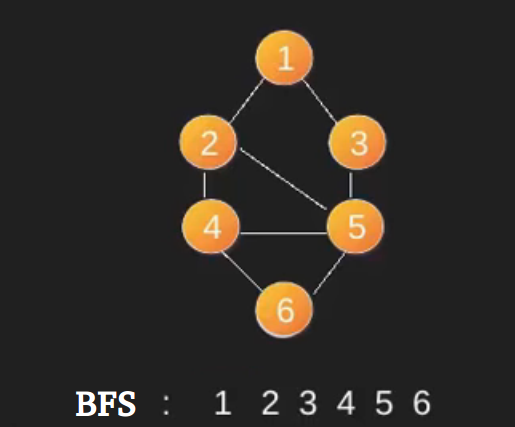GFG App
Open AppBrowser
Continue

# BFS using STL for competitive coding

A STL based simple implementation of BFS using queue and vector in STL. The adjacency list is represented using vectors of vector.

1. Create a queue and enqueue source into it.
1. Mark source as visited.
2. While queue is not empty, do following
1. Dequeue a vertex from queue. Let this  be f.
2. Print f
3. Enqueue all not yet visited adjacent of f and mark them visited.

Below is an example BFS starting from source vertex 1. Note that there can be multiple BFSs possible for a graph (even from a particular vertex).For more details of BFS, refer this post
The code here is simplified such that it could be used in competitive coding.

Implementation:

## CPP

 `// A Quick implementation of BFS using` `// vectors and queue` `#include ` `#define pb push_back`   `using` `namespace` `std;`   `vector<``bool``> v;` `vector > g;`   `void` `edge(``int` `a, ``int` `b)` `{` `    ``g[a].pb(b);`   `    ``// for undirected graph add this line` `    ``// g[b].pb(a);` `}`   `void` `bfs(``int` `u)` `{` `    ``queue<``int``> q;`   `    ``q.push(u);` `    ``v[u] = ``true``;`   `    ``while` `(!q.empty()) {`   `        ``int` `f = q.front();` `        ``q.pop();`   `        ``cout << f << ``" "``;`   `        ``// Enqueue all adjacent of f and mark them visited ` `        ``for` `(``auto` `i = g[f].begin(); i != g[f].end(); i++) {` `            ``if` `(!v[*i]) {` `                ``q.push(*i);` `                ``v[*i] = ``true``;` `            ``}` `        ``}` `    ``}` `}`   `// Driver code` `int` `main()` `{` `    ``int` `n, e;` `    ``cin >> n >> e;`   `    ``v.assign(n, ``false``);` `    ``g.assign(n, vector<``int``>());`   `    ``int` `a, b;` `    ``for` `(``int` `i = 0; i < e; i++) {` `        ``cin >> a >> b;` `        ``edge(a, b);` `    ``}`   `    ``for` `(``int` `i = 0; i < n; i++) {` `        ``if` `(!v[i])` `            ``bfs(i);` `    ``}`   `    ``return` `0;` `}`

```Input:
8 10
0 1
0 2
0 3
0 4
1 5
2 5
3 6
4 6
5 7
6 7

Output:
0 1 2 3 4 5 6 7```

Time Complexity: O(V+E) – we traverse all vertices at least once and check every edge.
Auxiliary Space: O(V) – for using a queue to store vertices.

This article is contributed by Nikhil Mahendran. If you like GeeksforGeeks and would like to contribute, you can also write an article using write.geeksforgeeks.org or mail your article to review-team@geeksforgeeks.org. See your article appearing on the GeeksforGeeks main page and help other Geeks.

My Personal Notes arrow_drop_up